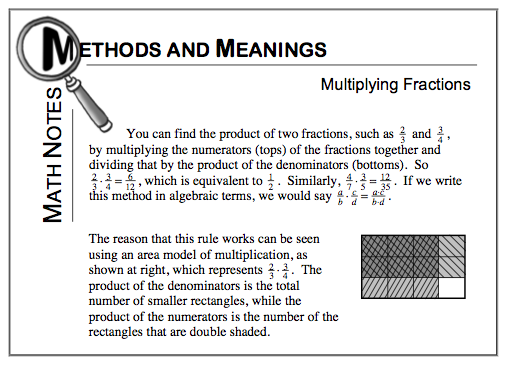### Home > MC1 > Chapter 7 > Lesson 7.2.3 > Problem7-73

7-73.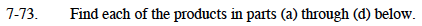$\left(\frac{2}{3}\right)\left(\frac{2}{7}\right)=\frac{(2)(2)}{(3)(7)}=\frac{4}{21}$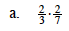Use the Math Notes box below if you are having trouble starting this problem.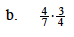Try drawing a picture to represent this problem, if you find that helpful.

$\frac{3}{7}$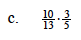Use the same method as in parts (a) and (b) to solve this problem.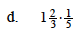You might want to make the mixed number into a fraction greater than one before you multiply.